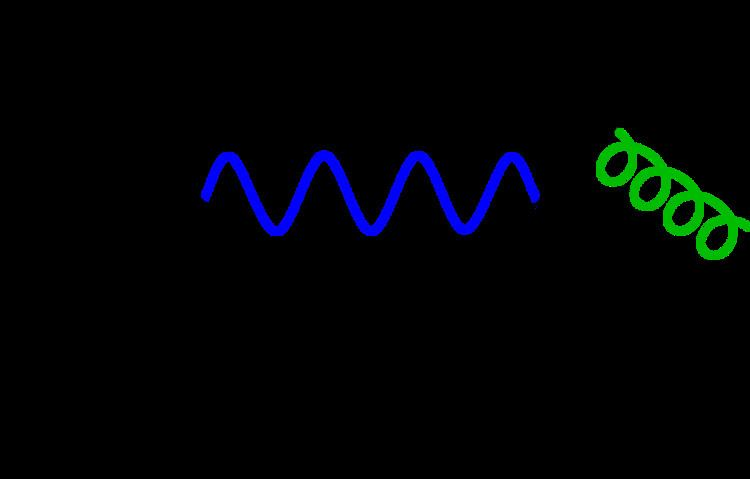# Quantization (physics)

Updated on
Covid-19In physics, quantization is the process of transition from a classical understanding of physical phenomena to a newer understanding known as quantum mechanics. It is a procedure for constructing a quantum field theory starting from a classical field theory. This is a generalization of the procedure for building quantum mechanics from classical mechanics. One also speaks of field quantization, as in the "quantization of the electromagnetic field", where one refers to photons as field "quanta" (for instance as light quanta). This procedure is basic to theories of particle physics, nuclear physics, condensed matter physics, and quantum optics.

## Quantization methods

Quantization converts classical fields into operators acting on quantum states of the field theory. The lowest energy state is called the vacuum state. The reason for quantizing a theory is to deduce properties of materials, objects or particles through the computation of quantum amplitudes, which may be very complicated. Such computations have to deal with certain subtleties called renormalization, which, if neglected, can often lead to nonsense results, such as the appearance of infinities in various amplitudes. The full specification of a quantization procedure requires methods of performing renormalization.

The first method to be developed for quantization of field theories was canonical quantization. While this is extremely easy to implement on sufficiently simple theories, there are many situations where other methods of quantization yield more efficient procedures for computing quantum amplitudes. However, the use of canonical quantization has left its mark on the language and interpretation of quantum field theory.

## Canonical quantization

Canonical quantization of a field theory is analogous to the construction of quantum mechanics from classical mechanics. The classical field is treated as a dynamical variable called the canonical coordinate, and its time-derivative is the canonical momentum. One introduces a commutation relation between these which is exactly the same as the commutation relation between a particle's position and momentum in quantum mechanics. Technically, one converts the field to an operator, through combinations of creation and annihilation operators. The field operator acts on quantum states of the theory. The lowest energy state is called the vacuum state. The procedure is also called second quantization.

This procedure can be applied to the quantization of any field theory: whether of fermions or bosons, and with any internal symmetry. However, it leads to a fairly simple picture of the vacuum state and is not easily amenable to use in some quantum field theories, such as quantum chromodynamics which is known to have a complicated vacuum characterized by many different condensates.

## Covariant canonical quantization

There is a way to perform a canonical quantization without having to resort to the non covariant approach of foliating spacetime and choosing a Hamiltonian. This method is based upon a classical action, but is different from the functional integral approach.

The method does not apply to all possible actions (for instance, actions with a noncausal structure or actions with gauge "flows"). It starts with the classical algebra of all (smooth) functionals over the configuration space. This algebra is quotiented over by the ideal generated by the Euler–Lagrange equations. Then, this quotient algebra is converted into a Poisson algebra by introducing a Poisson bracket derivable from the action, called the Peierls bracket. This Poisson algebra is then -deformed in the same way as in canonical quantization.

There is also a way to quantize actions with gauge "flows". It involves the Batalin–Vilkovisky formalism, an extension of the BRST formalism.

## Geometric quantization

In mathematical physics, geometric quantization is a mathematical approach to defining a quantum theory corresponding to a given classical theory. It attempts to carry out quantization, for which there is in general no exact recipe, in such a way that certain analogies between the classical theory and the quantum theory remain manifest. For example, the similarity between the Heisenberg equation in the Heisenberg picture of quantum mechanics and the Hamilton equation in classical physics should be built in.
One of the earliest attempts at a natural quantization was Weyl quantization, proposed by Hermann Weyl in 1927. Here, an attempt is made to associate a quantum-mechanical observable (a self-adjoint operator on a Hilbert space) with a real-valued function on classical phase space. The position and momentum in this phase space are mapped to the generators of the Heisenberg group, and the Hilbert space appears as a group representation of the Heisenberg group. In 1946, H. J. Groenewold considered the product of a pair of such observables and asked what the corresponding function would be on the classical phase space. This led him to discover the phase-space star-product of a pair of functions. More generally, this technique leads to deformation quantization, where the ★-product is taken to be a deformation of the algebra of functions on a symplectic manifold or Poisson manifold. However, as a natural quantization scheme (a functor), Weyl's map is not satisfactory. For example, the Weyl map of the classical angular-momentum-squared is not just the quantum angular momentum squared operator, but it further contains a constant term 3ħ2/2. (This extra term is actually physically significant, since it accounts for the nonvanishing angular momentum of the ground-state Bohr orbit in the hydrogen atom. As a mere representation change, however, Weyl's map underlies the alternate Phase space formulation of conventional quantum mechanics.

## Loop quantization

See Loop quantum gravity.

## Path integral quantization

A classical mechanical theory is given by an action with the permissible configurations being the ones which are extremal with respect to functional variations of the action. A quantum-mechanical description of the classical system can also be constructed from the action of the system by means of the path integral formulation.

## Quantum statistical mechanics approach

See Uncertainty principle

## Schwinger's variational approach

See Schwinger's quantum action principle

Quantization (physics) Wikipedia

Topics